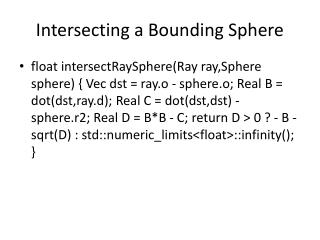# Intersecting a Bounding Sphere - PowerPoint PPT PresentationDownload PresentationIntersecting a Bounding Sphere

Download Presentation## Intersecting a Bounding Sphere

- - - - - - - - - - - - - - - - - - - - - - - - - - - E N D - - - - - - - - - - - - - - - - - - - - - - - - - - -
##### Presentation Transcript

1. Intersecting a Bounding Sphere • float intersectRaySphere(Ray ray,Sphere sphere) { Vecdst = ray.o - sphere.o; Real B = dot(dst,ray.d); Real C = dot(dst,dst) - sphere.r2; Real D = B*B - C; return D > 0 ? - B - sqrt(D) : std::numeric_limits<float>::infinity(); }

2. Quaternions

3. What are Quaternions? • An element of the 4-Dimensional vector-space

4. Why do you need quaternions? • No Gimbal Lock • They only require 4 numbers, to represent the equiv. of a 3x3 Matrix (9 Numbers) • Angle / Axis conversions are simple • Normalising Quaternions vs. Orthogonalising Matrices • Multiplicative like matrices

5. What a Quaternion looks like • w + xi + yj + zk (i, j, and k are imaginary numbers)